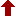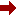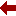#### SLIM21

 Sort Order Chronological Author Title ItemType,Author ItemType,Title Display Format Tabular Publication Bibliography Items / Page 5 10 25

Click the serial number on the left to view the details of the item.
#  AuthorTitleAccn#YearItem Type Claims
1 Kantorovich, Lev Mathematics for Natural Scientists I12528 2022 Book
2 Milstein, Grigori N Stochastic Numerics for Mathematical Physics I11924 2021 eBook
3 Saravanan, Rajendran Solvable One-Dimensional Multi-State Models for Statistical and Quantum Mechanics I11908 2021 eBook
 (page:1 / 1) [#3]

1.TitleMathematics for Natural Scientists : Fundamentals and Basics
Author(s)Kantorovich, Lev
PublicationCham, 1. Imprint: Springer 2. Springer International Publishing, 2022.
DescriptionXXIII, 768 p. 189 illus., 163 illus. in color : online resource
Abstract NoteThis book, now in a second revised and enlarged edition, covers a course of mathematics designed primarily for physics and engineering students. It includes all the essential material on mathematical methods, presented in a form accessible to physics students and avoiding unnecessary mathematical jargon and proofs that are comprehensible only to mathematicians. Instead, all proofs are given in a form that is clear and sufficiently convincing for a physicist. Examples, where appropriate, are given from physics contexts. Both solved and unsolved problems are provided in each section of the book. The second edition includes more on advanced algebra, polynomials and algebraic equations in significantly extended first two chapters on elementary mathematics, numerical and functional series and ordinary differential equations. Improvements have been made in all other chapters, with inclusion of additional material, to make the presentation clearer, more rigorous and coherent, and the number of problems has been increased at least twofold. Mathematics for Natural Scientists: Fundamentals and Basics is the first of two volumes. Advanced topics and their applications in physics are covered in the second volume the second edition of which the author is currently being working on
ISBN,Price9783030912222
Keyword(s)1. Applications of Mathematics 2. Chemometrics 3. Diseases 4. EBOOK 5. EBOOK - SPRINGER 6. ENGINEERING MATHEMATICS 7. Engineering???Data processing 8. Mathematical and Computational Engineering Applications 9. Mathematical Applications in Chemistry 10. Mathematical Methods in Physics 11. MATHEMATICAL PHYSICS 12. MATHEMATICS 13. Theoretical, Mathematical and Computational Physics
Item TypeBook
Circulation Data
 Accession# Call# Status Issued To Return Due On Physical Location I12528 On Shelf

2.TitleStochastic Numerics for Mathematical Physics
Author(s)Milstein, Grigori N;Tretyakov, Michael V
PublicationCham, Springer International Publishing, 2021.
DescriptionXXV, 736 p. 33 illus : online resource
Abstract NoteThis book is a substantially revised and expanded edition reflecting major developments in stochastic numerics since the first edition was published in 2004. The new topics, in particular, include mean-square and weak approximations in the case of nonglobally Lipschitz coefficients of Stochastic Differential Equations (SDEs) including the concept of rejecting trajectories; conditional probabilistic representations and their application to practical variance reduction using regression methods; multi-level Monte Carlo method; computing ergodic limits and additional classes of geometric integrators used in molecular dynamics; numerical methods for FBSDEs; approximation of parabolic SPDEs and nonlinear filtering problem based on the method of characteristics. SDEs have many applications in the natural sciences and in finance. Besides, the employment of probabilistic representations together with the Monte Carlo technique allows us to reduce the solution of multi-dimensional problems for partial differential equations to the integration of stochastic equations. This approach leads to powerful computational mathematics that is presented in the treatise. Many special schemes for SDEs are presented. In the second part of the book numerical methods for solving complicated problems for partial differential equations occurring in practical applications, both linear and nonlinear, are constructed. All the methods are presented with proofs and hence founded on rigorous reasoning, thus giving the book textbook potential. An overwhelming majority of the methods are accompanied by the corresponding numerical algorithms which are ready for implementation in practice. The book addresses researchers and graduate students in numerical analysis, applied probability, physics, chemistry, and engineering as well as mathematical biology and financial mathematics
ISBN,Price9783030820404
Keyword(s)1. BIOMATHEMATICS 2. Chemometrics 3. Computational Science and Engineering 4. EBOOK 5. EBOOK - SPRINGER 6. ENGINEERING MATHEMATICS 7. Engineering???Data processing 8. Mathematical and Computational Biology 9. Mathematical and Computational Engineering Applications 10. Mathematical Applications in Chemistry 11. MATHEMATICAL PHYSICS 12. Mathematics in Business, Economics and Finance 13. Mathematics???Data processing 14. Social sciences???Mathematics 15. Theoretical, Mathematical and Computational Physics
Item TypeeBook
Circulation Data
 Accession# Call# Status Issued To Return Due On Physical Location I11924 On Shelf

3.TitleSolvable One-Dimensional Multi-State Models for Statistical and Quantum Mechanics
Author(s)Saravanan, Rajendran;Chakraborty, Aniruddha
PublicationSingapore, Springer Nature Singapore, 2021.
DescriptionXIX, 174 p. 94 illus., 44 illus. in color : online resource
Abstract NoteThis book highlights the need for studying multi-state models analytically for understanding the physics of molecular processes. An intuitive picture about recently solved models of statistical and quantum mechanics is drawn along with presenting the methods developed to solve them. The models are relevant in the context of molecular processes taking place in gaseous phases and condensed phases, emphasized in the introduction. Chapter 1 derives the arisal of multi-state models for molecular processes from the full Hamiltonian description. The model equations are introduced and the literature review presented in short. In Chapter 2, the time-domain methods to solve Smoluchowski-based reaction-diffusion systems with single-state and two-state descriptions are discussed. Their corresponding analytical results derive new equilibrium concepts in reversible reactions and studies the effect of system and molecular parameters in condensed-phase chemical dynamics. In Chapter 3, time-domain methods to solve quantum scattering problems are developed. Along side introducing a brand new solvable model in quantum scattering, it discusses transient features of quantum two-state models. In interest with electronic transitions, a new solvable two-state model with localized non-adiabatic coupling is also presented. The book concludes by proposing the future scope of the model, thereby inviting new research in this fundamentally important and rich applicable field
ISBN,Price9789811666544
Keyword(s)1. Chemometrics 2. EBOOK 3. EBOOK - SPRINGER 4. Mathematical Applications in Chemistry 5. MATHEMATICAL PHYSICS 6. MATHEMATICAL STATISTICS 7. Theoretical, Mathematical and Computational Physics
Item TypeeBook